# Visual Basic 2013 Lesson 18: The Math Functions

#### [Lesson 17] << [Contents] >>[Lesson 19]

In lesson 11, we have learned how to write codes in Visual Basic 2013 that perform mathematical operations. However, to carry out more complex mathematical calculations, we need to use the built-in math functions in Visual Basic 2013. There are numerous built-in mathematical functions in Visual Basic 2013 that we shall introduce them one by one in this lesson.

### 18.1 The Abs function

The Abs function returns the absolute value of a given number.
The syntax is

`Math. Abs (number)`

* The Math keyword here indicates that the Abs function belongs to the Math class.

Example 18.1

In this example, we add a text box control for the user to input his or her number and a label control to display the absolute value of the number. We use the Val function to convert text to a numeric value. Rename the textbox as TxtNum and the label as LblAbs.

#### The Code

```Private Sub BtnComp_Click(sender As Object, e As EventArgs) Handles BtnComp.Click
LblAbs.Text = Math.Abs(Val(TxtNum.Text))
End Sub
```

The output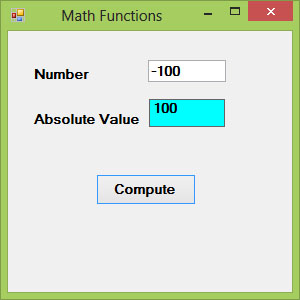Figure 18.1

### 18.2 The Exp function

The Exp function returns the exponential value of a given number. For example, Exp(1)=e=2.71828182

The syntax is

`Math.Exp (number)`

#### Example 18.2

In this example, we shall add a text box control for the user to input his or her number and a label control to display the exponential value of the number. Rename the textbox as TxtNum and the label as LblAbs.

#### The Code

```Private Sub BtnComp_Click(sender As Object, e As EventArgs) Handles BtnComp.Click
LblExp.Text = Math.Exp(Val(TxtNum.Text))
End Sub
```

The Output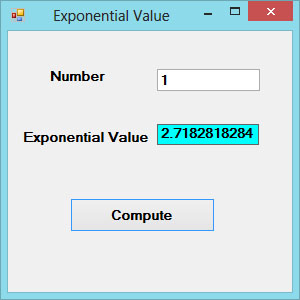Figure 18.2

### 18.3 The Fix Function

The Fix function truncates the decimal part of a positive number and returns the largest integer smaller than the number. However, when the number is negative, it returns the smallest integer larger than the number. Fix does not belong to the Math class, therefore, we do not use the Math keyword.

#### Example 18.3

```Private Sub BtnComp_Click(sender As Object, e As EventArgs) Handles BtnComp.Click
LblFixNum1.Text = Fix(Val(TxtPosNum.Text))
LblFixNum2.Text = Fix(Val(TxtNegNum.Text))
End Sub
```

The Output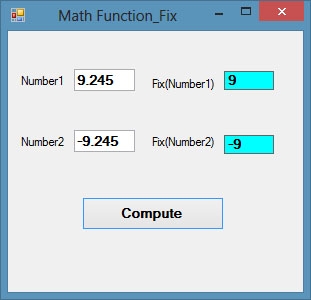Figure 18.3

### 18.4 The Int Function

The Int is a function that converts a number into an integer by truncating its decimal part and the resulting integer is the largest integer that is smaller than the number. For example

`Int(2.4)=2, Int(6.9)=6 , Int(-5.7)=-6, Int(-99.8)=-100`

### 18.5 The Log Function

The Log function is the function that returns the natural logarithm of a number.

Example 18.4

```Private Sub BtnComp_Click(sender As Object, e As EventArgs) Handles BtnComp.Click
LblLog.Text = Math.Log(Val(TxtNum.Text))
End Sub
```

The Output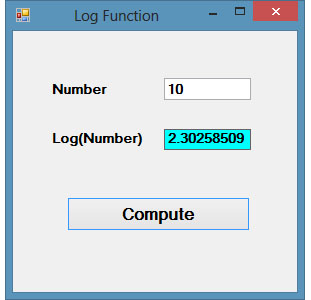Figure 18.4

### 18.6 The Rnd( ) Function

We use the Rnd function to write code that involves chance and probability. The Rnd function returns a random value between 0 and 1. Random numbers in their original form are not very useful in programming until we convert them to integers. For example, if we need to obtain a random output of 6 integers ranging from 1 to 6, which makes the program behave like a virtual dice, we can convert the random numbers to integers using the formula Int(Rnd*6)+1.

#### Example 18.5

```Private Sub BtnGen_Click(sender As Object, e As EventArgs) Handles BtnGen.Click
LblRnd.Text = Int(VBMath.Rnd() * 6) + 1
End Sub
```

Notice that the Rnd() function belongs to the VBMath class in Visual Basic 2013. This is different from Visual Basic 2012, where you can omit the VBMath keyword.

In this example, Int(VBMath.Rnd*6) will generate a random integer between 0 and 5. The function Int truncates the decimal part of the random number and returns an integer. After adding 1, you will get a random number between 1 and 6 every time you click the command button. For example, let say the random number generated is 0.98, after multiplying it by 6, it becomes 5.88, and using the integer function Int(5.88) will convert the number to 5, and after adding 1 you will get 6.

#### The Output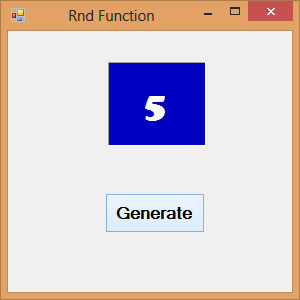Figure 18.5

*We shall learn  how to create an animated dice using a Timer control in later lesson

### 18.7 The Round Function

The Round function is the function that rounds up a number to a certain number of decimal places. The syntax is

`Round (n, m)`

which means to round a number n to m decimal places. For example,

`Math.Round (7.2567, 2) =7.26`

#### Example 18.6

```Private Sub Button1_Click(sender As Object, e As EventArgs) Handles Button1.Click
Label1.Text = Math.Round(Val(TextBox1.Text), 2)
End Sub
```

The Output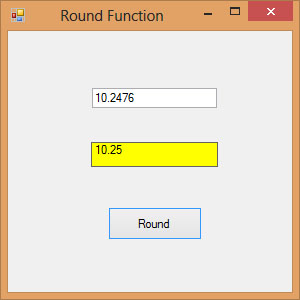Figure 18.6